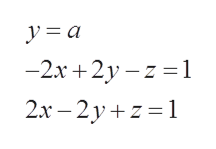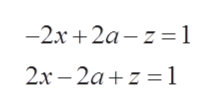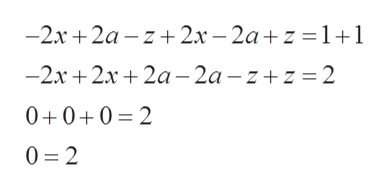Solve the system of linear equations in terms of a, where a = y.-2x+2y-z=12x-2y+z=1

Question
 Solve the system of linear equations in terms of a, where a = y. -2x+2y-z=1 2x-2y+z=1
Step 1

We are given system of equations ashelp_outlineImage Transcriptioncloseya -2x2 1 2x-2y 1 -z = ] Z fullscreen
Step 2

Firstly, we will plug back y=a into both equationshelp_outlineImage Transcriptionclose—2х + 2а-z %3D1 2х - 2а+z %3D1 fullscreen
Step 3

Now, we can add both equations

an...help_outlineImage Transcriptionclose-2x 2a z2x-2az 11 -2x 2x2a-2a -zz 2 0+0+0 2 0 2 fullscreen

Want to see the full answer?

See Solution

Want to see this answer and more?

Our solutions are written by experts, many with advanced degrees, and available 24/7

See Solution
Tagged in

Algebra